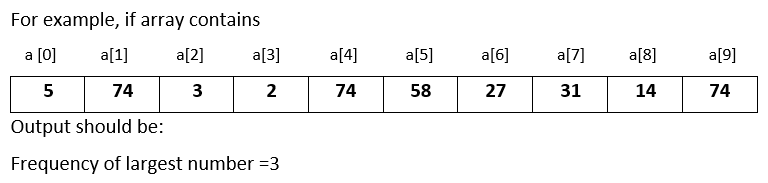# Input 10 numbers in 1d array and find the frequency of the largest number in C++

###### One Dimensional Array - Question 5

In this question, we will see how to input 10 numbers in a one dimensional integer array and find the frequency of the largest number in C++ programming. To know more about one dimensional array click on the one dimensional array lesson.

Q5) Write a program in C++ to input 10 numbers in a one dimensional integer array and find the frequency of the largest number.#### Program

``````#include <iostream>
#include <conio.h>

using namespace std;

int main()
{
int a, i,ln,c=0;

cout<<"Enter 10 numbers\n";
for(i=0; i<10; i++)
{
cin>>a[i];

if(i==0)
{
ln=a[i];
}
else if(a[i]>ln)
{
ln=a[i];
}
}

for(i=0; i<10; i++)
{
if(ln==a[i])
{
c++;
}
}

cout<<"Frequency of the Largest Number = "<<c;
return 0;
}``````

#### Output

```Enter 10 numbers
5
74
3
2
74
58
27
31
15
74
Frequency of the Largest Number = 3  ```